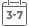# Nonparametric Regression Methods for Longitudinal Data Analysis: Mixed-Effects Modeling Approaches

• Publish Date: 2006-04-21
• Binding: Hardcover
• Author: Hulin Wu;Jin-Ting Zhang
• Sale
• \$217.51
• Regular price \$193.54Incorporates mixed-effects modeling techniques for more powerful and efficient methods

This book presents current and effective nonparametric regression techniques for longitudinal data analysis and systematically investigates the incorporation of mixed-effects modeling techniques into various nonparametric regression models. The authors emphasize modeling ideas and inference methodologies, although some theoretical results for the justification of the proposed methods are presented.

With its logical structure and organization, beginning with basic principles, the text develops the foundation needed to master advanced principles and applications. Following a brief overview, data examples from biomedical research studies are presented and point to the need for nonparametric regression analysis approaches. Next, the authors review mixed-effects models and nonparametric regression models, which are the two key building blocks of the proposed modeling techniques.

The core section of the book consists of four chapters dedicated to the major nonparametric regression methods: local polynomial, regression spline, smoothing spline, and penalized spline. The next two chapters extend these modeling techniques to semiparametric and time varying coefficient models for longitudinal data analysis. The final chapter examines discrete longitudinal data modeling and analysis.

Each chapter concludes with a summary that highlights key points and also provides bibliographic notes that point to additional sources for further study. Examples of data analysis from biomedical research are used to illustrate the methodologies contained throughout the book. Technical proofs are presented in separate appendices.

With its focus on solving problems, this is an excellent textbook for upper-level undergraduate and graduate courses in longitudinal data analysis. It is also recommended as a reference for biostatisticians and other theoretical and applied research statisticians with an interest in longitudinal data analysis. Not only do readers gain an understanding of the principles of various nonparametric regression methods, but they also gain a practical understanding of how to use the methods to tackle real-world problems.

MORE FROM THIS COLLECTION